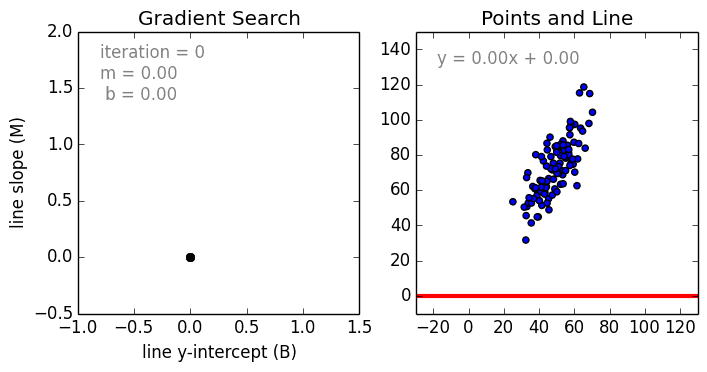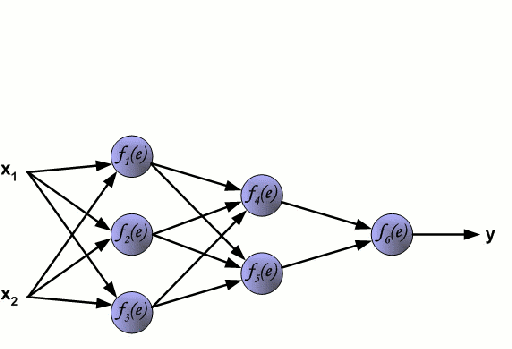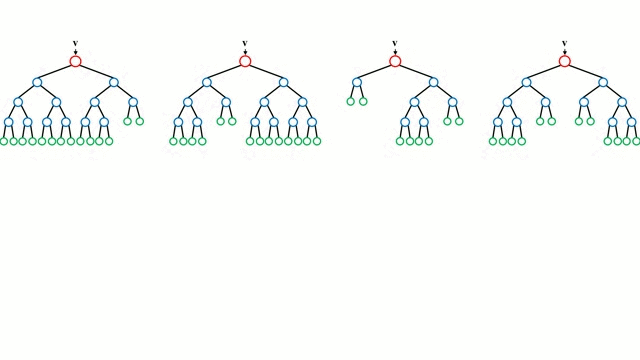# Selecting the Best Machine Learning Algorithm for Your Regression Problem

This post should then serve as a great aid in selecting the best ML algorithm for you regression problem!commentsWhen approaching any type of Machine Learning (ML) problem there are many different algorithms to choose from. In machine learning, there’s something called the “No Free Lunch” theorem which basically states that no one ML algorithm is best for all problems. The performance of different ML algorithms strongly depends on the size and structure of your data. Thus, the correct choice of algorithm often remains unclear unless we test out our algorithms directly through plain old trial and error.

But, there are some pros and cons to each ML algorithm that we can use as guidance. Although one algorithm won’t always be better than another, there are some properties of each algorithm that we can use as a guide in selecting the correct one quickly and tuning hyper parameters. We’re going to take a look at a few prominent ML algorithms for regression problems and set guidelines for when to use them based on their strengths and weaknesses. This post should then serve as a great aid in selecting the best ML algorithm for you regression problem!

### Linear and Polynomial RegressionLinear Regression

Beginning with the simple case, Single Variable Linear Regression is a technique used to model the relationship between a single input independent variable (feature variable) and an output dependent variable using a linear model i.e a line. The more general case is Multi Variable Linear Regressionwhere a model is created for the relationship between multiple independent input variables (feature variables) and an output dependent variable. The model remains linear in that the output is a linear combination of the input variables.

There is a third most general case called Polynomial Regression where the model now becomes a non-linear combination of the feature variables i.e there can be exponential variables, sine and cosine, etc. This however requires knowledge of how the data relates to the output. Regression models can be trained using Stochastic Gradient Descent (SGD).

Pros:

• Fast to model and is particularly useful when the relationship to be modeled is not extremely complex and if you don’t have a lot of data.
• Linear regression is simple to understand which can be very valuable for business decisions.

Cons:

• For non-linear data, polynomial regression can be quite challenging to design, as one must have some information about the structure of the data and relationship between feature variables.
• As a result of the above, these models are not as good as others when it comes to highly complex data.

### Neural NetworksNeural Network

A Neural Network consists of an interconnected group of nodes called neurons. The input feature variables from the data are passed to these neurons as a multi-variable linear combination, where the values multiplied by each feature variable are known as weights. A non-linearity is then applied to this linear combination which gives the neural network the ability to model complex non-linear relationships. A neural network can have multiple layers where the output of one layer is passed to the next one in the same way. At the output, there is generally no non-linearity applied. Neural Networks are trained using Stochastic Gradient Descent (SGD) and the backpropagation algorithm (both displayed in the GIF above).

Pros:

• Since neural networks can have many layers (and thus parameters) with non-linearities, they are very effective at modelling highly complex non-linear relationships.
• We generally don’t have to worry about the structure of the data at neural networks are very flexible in learning almost any kind of feature variable relationships.
• Research has consistently shown that simply giving the network more training data, whether totally new or from augmenting the original data set, benefits network performance.

Cons:

• Because of the complexity of these models, they’re not easy to interpret and understand.
• They can be quite challenging and computationally intensive to train, requiring careful hyper-parameter tuning and setting of the learning rate schedule.
• They require a lot of data to achieve high performance and are generally outperformed by other ML algorithms in “small data” cases.

### Regression Trees and Random ForestsRandom Forest

Beginning with the base case, a Decision Tree is an intuitive model where by one traverses down the branches of the tree and selects the next branch to go down based on a decision at a node. Tree induction is the task of taking a set of training instances as input, deciding which attributes are best to split on, splitting the dataset, and recurring on the resulting split datasets until all training instances are categorized. While building the tree, the goal is to split on the attributes which create the purest child nodes possible, which would keep to a minimum the number of splits that would need to be made in order to classify all instances in our dataset. Purity is measured by the concept of information gain, which relates to how much would need to be known about a previously-unseen instance in order for it to be properly classified. In practice, this is measured by comparing entropy, or the amount of information needed to classify a single instance of a current dataset partition, to the amount of information to classify a single instance if the current dataset partition were to be further partitioned on a given attribute.

Random Forests are simply an ensemble of decision trees. The input vector is run through multiple decision trees. For regression, the output value of all the trees is averaged; for classification a voting scheme is used to determine the final class.

Pros:

• Great at learning complex, highly non-linear relationships. They usually can achieve pretty high performance, better than polynomial regression and often on par with neural networks.
• Very easy to interpret and understand. Although the final trained model can learn complex relationships, the decision boundaries that are built during training are easy and practical to understand.

Cons:

• Because of the nature of training decision trees they can be prone to major overfitting. A completed decision tree model can be overly-complex and contain unnecessary structure. Though this can sometimes be alleviated using proper tree pruning and larger random forest ensembles.
• Using larger random forest ensembles to achieve higher performance comes with the drawbacks of being slower and requiring more memory.

### Conclusion

Boom! There’s your pros and cons! In the next post we’ll take a look at the pros and cons of different classification models. I hope you enjoyed this post and learned something new and useful. If you did, feel free to give it some claps.

Bio: George Seif is a Certified Nerd and AI / Machine Learning Engineer.

Original. Reposted with permission.

Related: